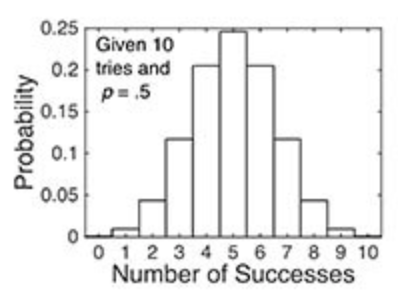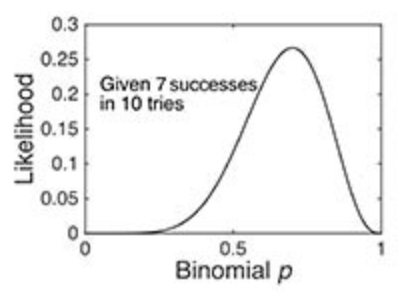# C. Randy Gallistel - «Bayes for Beginners: Probability and Likelihood» (2015)

## Distinguishing Likelihood From Probability

The distinction between probability and likelihood is fundamentally important:

• probability attaches to possible results;
• likelihood attaches to hypotheses.

Possible results are mutually exclusive and exhaustive.
Suppose we ask a subject to predict the outcome of each of 10 tosses of a coin.
There are only 11 possible results (0 to 10 correct predictions).
The actual result will always be one and only one of the possible results.
Thus, the probabilities that attach to the possible results must sum to 1.

Hypotheses, unlike results, are neither mutually exclusive nor exhaustive.
Suppose that the first subject we test predicts 7 of the 10 outcomes correctly.
I might hypothesize that the subject just guessed, and you might hypothesize that the subject may be somewhat clairvoyant, by which you mean that the subject may be expected to correctly predict the results at slightly greater than chance rates over the long run.
These are different hypotheses, but they are not mutually exclusive, because you hedged when you said “may be.”
You thereby allowed your hypothesis to include mine.
In technical terminology, my hypothesis is nested within yours.
Someone else might hypothesize that the subject is strongly clairvoyant and that the observed result underestimates the probability that her next prediction will be correct.
Another person could hypothesize something else altogether.
There is no limit to the hypotheses one might entertain.

The set of hypotheses to which we attach likelihoods is limited by our capacity to dream them up.
In practice, we can rarely be confident that we have imagined all the possible hypotheses.

Our concern is to estimate the extent to which the experimental results affect the relative likelihood of the hypotheses we and others currently entertain.

Because we generally do not entertain the full set of alternative hypotheses and because some are nested within others, the likelihoods that we attach to our hypotheses do not have any meaning in and of themselves; only the relative likelihoods — that is, the ratios of two likelihoods — have meaning.

## Using the Same Function ‘Forwards’ and ‘Backwards’

The difference between probability and likelihood becomes clear when one uses the probability distribution function in general-purpose programming languages.

In the present case, the function we want is the binomial distribution function.

It is called `BINOM.DIST` in the most common spreadsheet software and `binopdf` in the language I use.
It has three input arguments:

• the number of successes,
• the number of tries,
• the probability of a success.

When one uses it to compute probabilities, one assumes that the latter two arguments (number of tries and the probability of success) are given .
They are the parameters of the distribution.
One varies the first argument (the different possible numbers of successes) in order to find the probabilities that attach to those different possible results.
Regardless of the given parameter values, the probabilities always sum to 1.The binomial probability distribution function, given 10 tries at p = .5.The binomial likelihood function, given 7 successes in 10 tries.

Both panels were computed using the `binopdf` function.

• In the first panel, I varied the possible results.
• In the second panel, I varied the values of the p parameter.
• The probability distribution function is discrete because there are only 11 possible experimental results (hence, a bar plot).
• By contrast, the likelihood function is continuous because the probability parameter p can take on any of the infinite values between 0 and 1.
• The probabilities in the first plot sum to 1.
• The integral of the continuous likelihood function in the bottom panel is much less than 1; that is, the likelihoods do not sum to 1.

psychologicalscience.org/observer/bayes-for-beginners-probability-and-likelihood